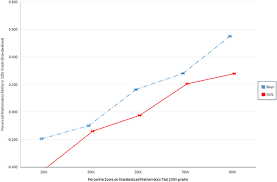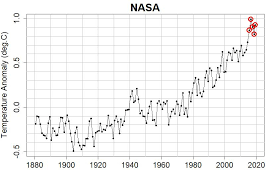## How to Calculate and Solve for TEX | Denier | Polymer & TextileThe image above represents TEX | Denier.

To compute for TEX | Denier, one essential parameter is needed and this parameter is denier (D).

The formula for calculating the TEX | Denier:

TEX = Denier / 9

Where;

TEX = TEX
D = Denier

Let’s solve an example;
Given that denier is 12. Find the TEX | Denier?

This implies that;

D = Denier = 12

TEX = Denier / 9
TEX = 12 / 9
TEX = 1.33

Therefore, the TEX is 1.33.

## How to Calculate and Solve for Denier | Polymer & TextileThe image above represents denier.

To compute for denier, two essential parameters are needed and these parameters are mass (M) and length (L).

The formula for calculating denier:

Denier = M/L x 9000

Where;

D = Denier
M = Mass
L = Length

Let’s solve an example;
Find the denier with a mass of 24 and a length of 28.

This implies that;

M = Mass = 24
L = Length = 28

Denier = M/L x 9000
Denier = 24/28 x 9000
Denier = 0.857

Therefore, the denier is 0.857.

Calculating the Mass when the Denier and the Length is Given.

M = DL / 9000

Where;

M = Mass
D = Denier
L = Length

Let’s solve an example;
Find the mass of a denier with 30 and a length of 18.

This implies that;

D = Denier = 30
L = Length = 18

M = DL / 9000
M = 30 x 18 / 9000
M = 540 / 9000
M = 0.06

Therefore, the mass is 0.06.